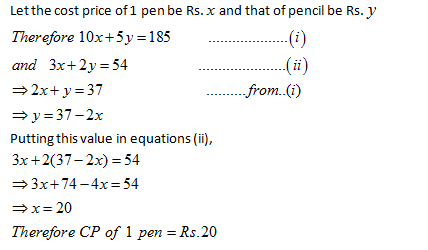• This is an assessment test.
• These tests focus on the basics of Maths and are meant to indicate your preparation level for the subject.
• Kindly take the tests in this series with a pre-defined schedule.

## Basic Maths: Test 49

Congratulations - you have completed Basic Maths: Test 49.

You scored %%SCORE%% out of %%TOTAL%%.

Your performance has been rated as %%RATING%%

 Question 1
Garima bought 10 pens, 12 packets of wax colours, 5 calculators and 8 pencil boxes, The price of one pen is Rs.8, one packet of wax colour is Rs.25, one calculator is Rs.170 and one pencil box is Rs.15 more than the combined price of one pen and one packet of wax colours. How much amount did harshita pay to the shopkeeper?
 A Rs.1681 B Rs.1814 C Rs.1614 D Rs.1672
Question 1 Explanation:
\begin{align} & C.P\,\,\,of\,\,one\,\,\,pencil\,\,\,box\, \\ & =8+25+15=Rs.\,48 \\ & Total\,\,amount\,\,paid\,\,by\,\,Garima \\ & =Rs.\,\left( 10\times 8+12\times 25+5\times 170+8\times 48 \right) \\ & =Rs.\,\left( 80+300+850+384 \right) \\ & =Rs.\,1614 \\ \end{align}
 Question 2
${{\left( 2626 \right)}^{2}}=?\div 2626$
 A 1/2626 B (2626)3 C 2626 D (2626)3/2
Question 2 Explanation:
\begin{align} & {{\left( 2626 \right)}^{2}}=?\div 2626 \\ & \Rightarrow ?={{\left( 2626 \right)}^{2}}\times 2626 \\ & =2626\times 2626\times 2626 \\ & ={{\left( 2626 \right)}^{3}} \\ \end{align}
 Question 3
Boman’s present age is three times his daughter's present age and nine-thirteenth of his mother's present age. The sum of the present ages of all of them is 125 years. What is the difference between the Boman's daughter's present age and Boman's mother's present age?
 A 45 years B 40 years C 50 years D Cannot be determined
Question 3 Explanation:
Let Boman’s present age = $x$ year
Therefore His daughter’s present age = $\frac{x}{3}$years
His mother’s present age = $\frac{13x}{9}$years
\begin{align} & Therfore\,\,x+\frac{x}{3}+\frac{13x}{9}=125 \\ & \Rightarrow \frac{9x+3x+13x}{9}=125 \\ & \Rightarrow 25x=125\times 9 \\ & \Rightarrow x=\frac{125\times 9}{25}=45 \\ & Therefore\,\,\operatorname{Re}quired\,\,difference \\ & =\frac{13x}{9}-\frac{x}{3} \\ & =\frac{13x-3x}{9}=\frac{10x}{9} \\ & =\frac{10}{9}\times 45=50\,\,years \\ \end{align}
 Question 4
Cost of 10 pens and 5 pencils is Rs.185 and the cost of 3 pens and 2 pencils Is Rs.54. What is the cost of one pen?
 A Rs.16 B Rs.14 C Rs.12 D None
Question 4 Explanation:Question 5
Cost of 21 shirts and 14 trousers Is Rs.21,000. What will be the cost of 30 shirts and 20 trousers?
 A Rs.30,500 B Rs.30,000 C Rs.35,000 D Cannot be determined
Question 5 Explanation:
Let the cost of 1 shirt be Rs.$x$
And that of 1 trouser be Rs. $y$
\begin{align} & Therefore\,\,21x+14y=21000 \\ & \Rightarrow \,3x+2y=3000\,\,\,\,\,\,\,\,\,\,\,\,\,\,\,\,..............\left( i \right) \\ \end{align}
Multiplying both sides by 10,
30x + 20y = 30000
Once you are finished, click the button below. Any items you have not completed will be marked incorrect.
There are 5 questions to complete.
 ← List →
Shaded items are complete.
 1 2 3 4 5 End